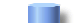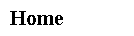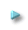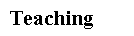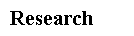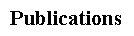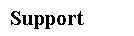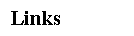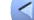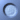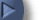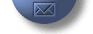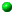Courses Taught
• STA 2171: Statistics for Biology
This course provides an introduction to statistics emphasizing applications to biology. Topics include: descriptive statistics, elementary probability, the binomial and normal distributions, confidence intervals and hypothesis tests for means and proportions, correlation and regression, contingency tables and goodness-of-fit tests as well as analysis of variance. Software: Minitab (Optional).

• STA 3032: Applied Statistics for Scientists and Engineers
This course will cover calculus-based probability, discrete and continuous random variables, joint distributions, and sampling distributions. Topics include descriptive statistics, interval estimates and hypothesis tests, ANOVA, correlation, simple and multiple regression, analysis of categorical data, and statistical quality control. Software: Minitab (Optional).

• STA 4102/5106: Computational Methods in Statistics I
This course provides an introduction to the commonly-used computaional methods in statistics. The students are expected to gain an understanding of the techniques and ideas used in implementing mathematical/statistical formulations on computers. Topics inlcude Linear Methods for Regression Analysis, Nonlinear Methods for Maximum Likelihood Estimation, Elementary Pattern Recognition, Simulation of Random Variables, Monte-Carlo methods for Integration, and Dynamic Programming. Software: Matlab, Python.

• STA 4103/5107: Computational Methods in Statistics II
This course is a continuation of STA4102/5106. More advanced computational methods in statistics will be introduced and discussed. Topics inlcude Markov Chain Monte Carlo Methods, Analysis of Dynamic Systems, Sequential Monte Carlo Method, Bootstrap Methods, Simulation of Stochastic Processes, Point Process Models, Stochastic Optimization, and Statistics on Nonlinear Spaces. Software: Matlab, Python.

• STA 4203/5207: Applied Regression Methods
This course provides an introduction to the basic principles of regression. Topics include general linear hypothesis, analysis of covariance, multiple correlation and regression, response surface methods. Software: R.

• STA 4321/5323: Introduction to Mathematical Statistics
Students will learn some basic concepts and methods in mathematical statistics. This course focuses on topics including basic probability theory, counting methods, conditional probability, random variables, discrete and continuous distributions, bivariate distribution, expectation, variance, covariance, and correlation.

• STA 4853/5856: Time Series and Forecasting Methods
This course discusses a class of linear time series models that include autoregressive models, moving-average models, ARIMA models, and dynamic regression models. The models have been widely applied to data in many fields of social, biological and physical sciences. Topics include autoregressive, moving average and mixed models, autocovariance and autocorrelation functions, model identification, forecasting techniques, seasonal model identification estimation and forecasting, intervention and transfer function model identification, estimation and forecasting. Software: SAS.

• STA 6246: Statistical Methods in Neuroscience
This course provides an introduction to the classical and recent statistical methods used in the field of neuroscience. Topics inlcude Properties of Neurons, Probability Models, Correlograms, Regressions, Wiener filter, LMS, RLS, Newton-Raphson Method, Kalman filter, extended Kalman filter, Kalman smoother, MCMC, particle filter, EM-algorithm, mixture model, switching Kalman filter, HMM, Dynamic programming, State-space models, point process filter, and Laplace approximation. Software: Matlab.